Home > A2C > Chapter 11 > Lesson 11.2.5 > Problem11-105

11-105.
1. Use properties of exponents to rewrite each expression below so that it involves only multiplication and exponents. Homework Help ✎

1.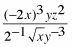2.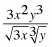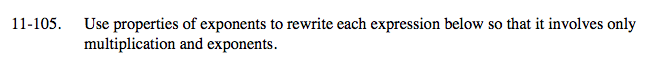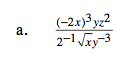$16\,x^{ \frac{5}{2}}\,\,y^{4}\,z^{3}$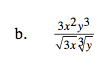$3^{\frac{1}{2}}\,\,x^{\frac{3}{2}}\,\,y^{\frac{8}{3}}$